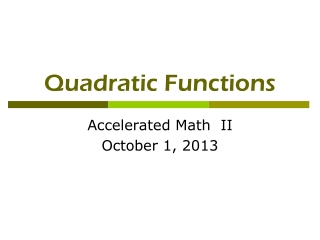DownloadDownload PresentationTélécharger la présentation- - - - - - - - - - - - - - - - - - - - - - - - - - - E N D - - - - - - - - - - - - - - - - - - - - - - - - - - -
Presentation Transcript

1. Quadratic Functions Accelerated Math II October 1, 2013

2. One-Minute Question If f(x) = 3x2 + 6x, state the vertex of f(x).

3. One-Minute Question If f(x) = 3x2 + 6x, state the vertex of f(x). x= and f(-1) =3 – 6 = -3, so (-1, -3)

4. What would you put on a test covering quadratic functions? How do you find their roots? Factoring Completing a square Quadratic formula

5. What would you put on a test covering quadratic functions? How do you find the x values of their vertices? Average their roots Completing a square Calculate –b/(2a)

6. What would you put on a test covering quadratic functions? How do you find the y value of their vertices (maximum or minimum value)? Completing a square Calculate f(-b/(2a))

7. What would you put on a test covering quadratic functions? What are different forms of a quadratic function? Standard: y = ax2 + bx + c Vertex: y = a(x – h)2 + k Factored or intercept : y = a(x – r1)(x – r2)

8. What would you put on a test covering quadratic functions? Can you switch from form to form algebraically??

9. How do you graph a quadratic function? • y = x2 • y = x2 + 4 • y = (x + 3)2 • y = 2(x – 3)2 + 5 • What’s the vertex of y = a(x – h)2 + k ?

10. What would you put on a test covering quadratic functions? What affects the shape of their graph? “a” vertically stretches or shrinks or inverts. “h” translates horizontally. “k” translates vertically.

11. What would you put on a test covering quadratic functions? What equation would produce this graph?

12. How do you solve quadratic equations? • X2 + 15 = 79 • (X + 4)2 - 14 = 11 • X2 + 6x = 7 • 2x2 = 7x + 15 • Find the roots of f(x) = x2 + 2x – 35

13. How do you solve harder quadratic equations? • X2 + 15 = 9 • (X + 4)2 - 10 = 11 • X2 + 6x = 5 • 2x2 = 7x + 10 • Find the roots of f(x) = 3x2 + 2x – 35

14. How do you solve harder quadratic inequalities? • X2 + 18 > 9x • (X + 4)2 - 10 < 15 • X2 + 6x < 5 • y > 2x2 - x - 10 • y < -x2 + x + 12

15. How do you solve systems of quadratics? Y = x2 + 3x – 7 Y = 2x2 - 5

16. APPLICATIONS!! • See book…

17. Logic about Quadratics • IF f(x) = 2x2 + kx – 20 has one root at 4, find its other root.